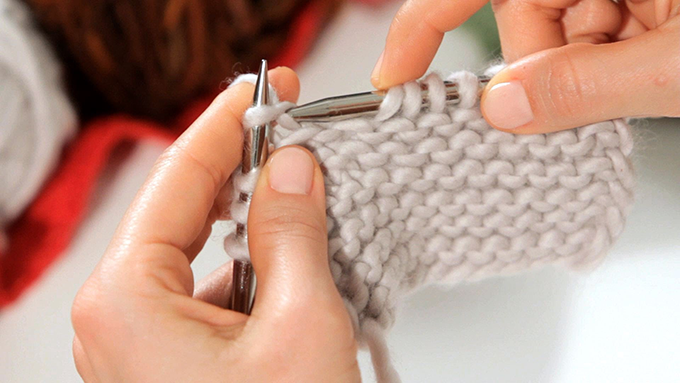# Why I use knitting to teach mathShare:On a snowy day in January, I asked students to tell me what was the first word that came to mind when thinking about mathematics. The two most quoted words were “calculus” and “equation”. When I asked the same question to a room full of professional mathematicians, none of these two words were spoken; they spoke to me about “critical thinking” and “problem solving”. The experience is not very surprising. What professional mathematicians consider to be mathematics has nothing to do with the image of the general population. While so many people refer to mathematics as a calculus-only discipline, it’s no wonder we hear so often, “I hate math.” So I decided to take this question in an unconventional way, by offering a course entitled “Mathematics of Knitting” in my school, Carthage College. During the sessions, I chose to eliminate all use of pencils, papers, calculators (goups!) And textbooks. Instead, we have discussed, done handicrafts, drawn and played with all kinds of objects – beach balls or sewing meters, for example. As personal homework, we nourished our thinking by holding a common blog. And of course, we knitted.

#### Equations in question

The equation is at the heart of mathematics; and in an equation, the equal sign plays a crucial role. An equation like x = 5 tells us that the dreaded x, which represents a certain quantity, has the same value as 5. The number 5 and the value of x must therefore be exactly the same. The meaning of the equal sign is very strict. That there is the slightest sprain in the word “exactly”, and that means that the two things put in equation are not equal. However, it often happens in everyday life that two quantities, without being exactly the same, are equivalent to other criteria. Imagine, for example, that you have two square cushions. The first is red on the top, yellow on the right side, green on the bottom and blue on the left. The second is yellow at the top, green at the right side, blue at the bottom and red at the left. These cushions are not exactly the same. One has a red top, the other has a yellow one. But they are similar, without a shadow of a doubt. In fact, they would be exactly the same if you applied to the cushion with the red vertex a simple rotation in the opposite direction of the clockwise. How many different ways can I have the same colored cushion on a bed so that it looks different each time? A small exercise shows that there are 24 configurations of possible patterns with the same color scheme, eight of which are observable by moving the cushion. The students experienced this by knitting cushions made of two colors from knitting diagrams. Students created square knitting tables in which the eight movements in the painting each gave a different picture. These images were then knitted in a cushion where their equivalence could be demonstrated by actually moving the cushion.

#### Geometry and rubber

Another topic we have discussed is what is called “rubber geometry”. It is a question of imagining that the whole world is made of rubber, then to transpose this idea in the form of geometrical figures. Let’s try to understand the concept with knitting. To knit round objects – such as hats or gloves – special knitting needles, called double-pointed needles, can be used. During manufacture, the hat is shaped by three needles, which gives it a triangular shape. Then, once the needles are removed, the stretchable thread relaxes to form a circle, and the characteristic shape of the hat is recognized. This is the concept that “rubber geometry” tries to capture. In a way, a triangle and a circle may be identical if they are made of a flexible material. In fact, in this area of ​​study, all polygons become circles. But if all polygons are circles, what are the forms that allow them to differentiate? There are some traits that stand out even when the objects are flexible – for example, whether a shape has edges or not, holes or not, includes twists or not. The infinite scarf, for example, is not equivalent to a circle. To create an “infinite paper scarf” at home, take a long strip of paper and paste its shortest short edges by matching the upper left corner with the lower right corner and the lower left corner with the upper right corner. Then draw arrows pointing all along the object. You should observe an amazing and friendly phenomenon. Students in my class spent time knitting objects, such as scarves and infinity headbands, that showed differences, even when made of soft material. The addition of markers – like arrows – made it possible to visualize precisely how the objects differed.

#### Multiple flavors

If what I am talking about here seems to you to be unrelated to mathematics, I insist that it is the case. The issues discussed in this paper – abstract algebra and topology – are topics that are discussed at the university when studying mathematics. But their stakes are accessible to all, if indeed we have the right mediation tools. In my opinion, these different flavors of mathematics should not be hidden from the general public or less emphasized than conventional mathematics. In addition, studies have shown that the use of physically manipulable material can enhance mathematical learning at any level of education. If more mathematicians were able to take a step aside in traditional teaching techniques, it would be possible to defeat this misconception but widespread belief that mathematics is reduced to calculation. And maybe more people would be seduced by mathematical reasoning. Author Bio: Sara Jensen is Assistant Professor of Mathematics at Carthage College

Tags: